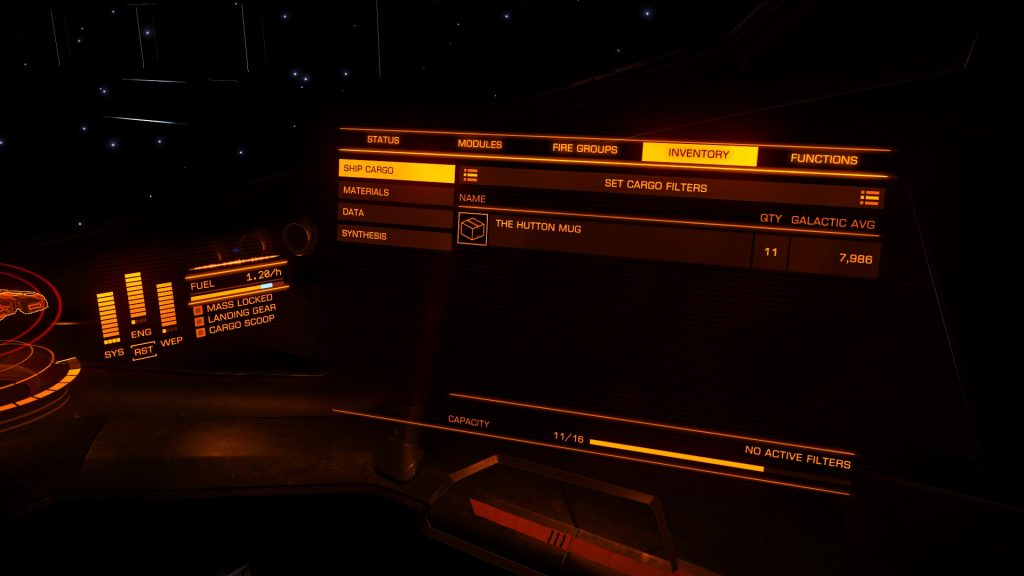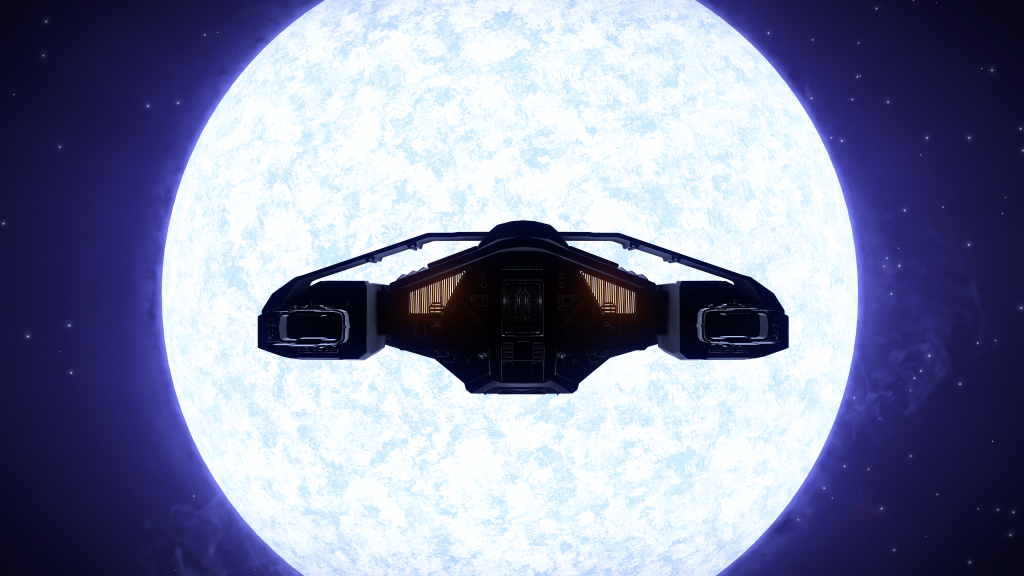# Jaques – 25th Sep 3302 (Day 15)

Today, I started off by going for a wonder in my SRV around a high metal content planet in the system I left off in – Clooku YS-D b43-3. After finding some materials – including the Vanadium I needed for my AFMU – I went back to my ship to continue onwards. This was the highest-gravity world I’ve been on so far, at ~1.3G. Apparently, this makes it easier to flip my SRV onto it’s roof… although I’m fairly sure the really rough terrain does not help.

When I launched my ship, I decided I’d see what materials I now had, and discovered (to my dismay) that I’ve lost five of my sixteen mugs, so I’m down to 11 remaining. Having said this, I noticed yesterday that the jump range on the galaxy map was mysteriously set to 11/16, so this is probably why. One of the components that did malfunction during that 200% overheat incident was the cargo hatch, so I strongly suspect that this is what has caused me to lose some of those mugs.En-route to Waypoint 15, I discovered a very pretty B-class star, with a really intense blue glow that was just stunning. Even the glow on the console in front of me was impressive as I was passing it.Anyway, I’ve reached Waypoint 15, which (I believe) puts me ahead of CMDR Braxton Doyle, whose waypoints I was following. Thus, this session has mostly been taken up by me trying to figure out how to plot the next waypoint. The rough process I’ve taken is this:

1. Get the coordinates of Jaques (-9530.5, -910.28125, 19808.125) and my current location at Waypoint 15 (Stuelou DT-T b20-2; -6524.1875, -622.28125, 13460.53125), and calculate the distance between the two points:$latex x = -3006.3125, y = -288, z = 6347.59375$
2. Use Pythagoras’ Theorem to calculate the direct distance $latex h$ between the two points:h = \sqrt{x^2 + y^2 + z^2} = 7029.424
3. Let the maximum rangefinder distance h' = 1000
4. Let $latex (x,y,d)$ be a right-angled triangle, and $latex (z,h,d)$ be a seconds right angled triangle, the normals of which are perpendicular, such that $latex d$ becomes the hypotenuse of the $latex (x,y)$ pair, and $latex h$ represents the entire vector from WP15 to Jaques.
5. Calculate the angle $latex \theta$ adjacent to the $latex x$ and the hypotenuse completing the triangle with the Y axis: \theta = \tan ^{-1} \frac{y}{x} = 0.095507
6. Calculate the angle $latex \theta’$ adjacent to $latex h$ and the hypotenuse completing the triangle with the Y axis: \theta' = \sin^{-1} \frac{z}{h} = 1.126709
7. z' = h' \sin{\theta'} = 903.0034
8. d' = \sqrt{h'^2 - z'^2} = 429.6335
9. x' = d' \cos \theta = 427.675
10. y' = \sqrt{d'^2 - x'^2} = 40.9706
11. This gives $latex (\pm x’, \pm y’, \pm z’)$, a directionless vector, which now needs to be applied to your current postion to give a set of coordinates. Note that each component may have a different direction. In my case, the X and Y components were negative, and the Z component was positive.
12. Push steps 1 through 10 into an Excel spreadsheet for future use
13. Take as the maximum coordinates of the next waypoint, and go to those coordinates on the galaxy map, choosing the closest system to that point that is less than 1000ly from our current position. I don’t know a way of automating this yet, especially given places like EDSM are incomplete.

From the looks of it on the Galaxy Map, the waypoint looks approximately correct, so I’m going to take this process as Good Enough™, and use it going forward.

I still haven’t got the hang of not flying into stars it would seem – straight after an overheating incident caused by me staying too close for too long while scooping, I decided it would be smart to fly close to a Y-class brown dwarf, causing my ship to nope out and emergency stop out of supercruise..

Now I’m at Waypoint 16, I think it’s time for me to plot Waypoint 17, then go to bed. Clearly, I’m too unsafe to fly now!

## Statistics

 Current location Blua Eaec BR-B a6-1 Next waypoint Blua Eaec VW-Y b44-16 (999.77 ly, 62bcj) Distance from Colonia 6031.77 ly (373 bcj) Incident count 6 Jumps made 129 Jumps remaining (best case) 373 Space madness 64 %

### Incidents

 Unsafe drop from Supercruise (into star) 1 Overheat 1 Flipped SRV onto roof 4